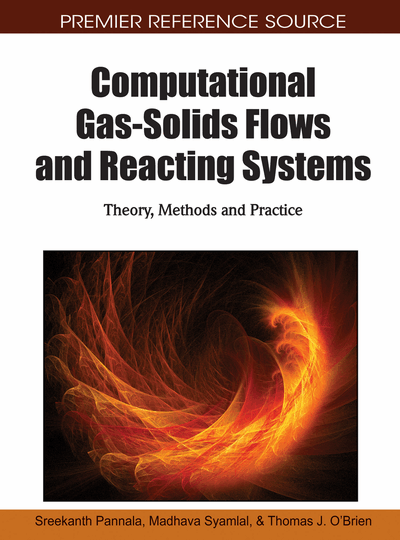# The Multiphase Particle-in-Cell (MP-PIC) Method for Dense Particle Flow

Dale M. Snider (CPFD, USA) and Peter J. O’Rourke (CPFD, USA)
DOI: 10.4018/978-1-61520-651-3.ch009
Available
\$37.50
No Current Special Offers

## Abstract

This chapter is a review of the multiphase particle-in-cell (MP-PIC) numerical method for predicting dense gas-solids flow. The MP-PIC method is a hybrid method such as IBM method described in the previous chapter, where the gas-phase is treated as a continuum in the Eulerian reference frame and the solids are modeled in the Lagrangian reference frame by tracking computational particles. The MP-PIC is a derivative of the Particle-in-Cell (PIC) method for multiphase flows and the method employs a fixed Eulerian grid, and Lagrangian parcels are used to transport mass, momentum, and energy through this grid in a way that preserves the identities of the different materials associated with the particles. The main distinction with traditional Eulerian-Lagrangian methods is that the interactions between the particles are calculated on the Eulerian grid. One of the main advantages of PIC methods is the accuracy of the convection algorithms (Lagrangian advection is non-diffusive) and this can be important aspect of gas-solids flows where the overall dynamics is dictated by the instabilities in the system due to sharp interfaces between the phases. Additional details about this method along with examples are provided in this chapter. In the following chapter, the application of this method to Circulating Fluidized Beds (CFBs) is described.
Chapter Preview
Top

## Introduction

For the most part, mathematical models of separated particulate multiphase flow have used either a continuum approach for both phases (Gidaspow, 1986, Batchelor, 1988, Jackson, 2000) or a continuum approach for the fluid and a Lagrangian computational-particle model for particle phase (Cundall & Strack, 1979, Amsden, et al,, 1989, Snider 2001, Godlieb, et al, 2007). The two-fluid continuum approach averages both fluids and solids by a statistical procedure (typically ensemble-averaging) and treats the solids phase as a pseudo continuum. The averaging procedure leads to many unclosed terms which must be modeled (Jackson, 2000), and much progress has been made in the modeling of such terms as the particle-particle-stresses and particle viscous-stresses in dense particle flows using spatial gradients of average particle properties (Batchelor, 1988, Gidaspow, 1994).

The two-fluid continuum approach has trouble modeling flows with a distribution of particle types and sizes because separate continuity and momentum equations must be solved for each size and type (Gidaspow, 1994, Risk, 1993). While a continuous-fluid description of the solids phase has application in some solid-fluid flow regimes it is inaccurate in others. For dilute solid flows, closure models based on the assumption of high collision frequencies are questionable. In addition, the non-linear behavior of some solid flows is difficult to model with a Navier-Stokes type momentum equation. The different characters of fluid and solid flows are seen in an hour-glass and in a U-tube. The hour glass works as a useful measure of time because the particle flow rate through the orifice is constant and independent of sand depth. If instead, a fluid were used in the hour glass, the flow rate is initially high because of a hydrostatic head, and the flow tapers off as the hour glass empties of fluid. In the simple U-tube, a fluid will fill both arms to the same height (manometer). However, solids poured into one arm of the U-tube will only fill the one arm.

Modeling the particle phase as discrete particles or elements has a number of variations. The basic direct numerical solution of resolving the fluid flow around each particle with no-slip condition, and coupling the motion of particles, can only be done for a small number of particles. Similarly, Lattice Boltzmann calculations are limited to a small number of particles. Both methods are useful in studying fundamental solid-fluid flow behavior and can be used in developing closure models for fluid-particle interactions needed in continuum models. By averaging the fluid over a spatial region containing a number of particles and using a closure model for the fluid-particle momentum transfer, large systems can be modeled. The discrete particle or discrete element method (DPM or DEM) (Cundal & Strack, 1979) is based on a finite number of discrete semi-rigid spherically, or regularly, shaped particles interacting through contact forces and transferring momentum to and from the fluid by a drag closure model. The multitude of particle-to-particle contacts and the model for the contact force are the most significant parts of the direct element model. To efficiently calculate particle interactions, relatively simple models, often based on a simple coefficient of restitution, are employed. DPM allows solution for flows with a wide range of particle types, sizes, shapes and velocities. Because, of the high collision frequency for volume fractions above 5% (O'Rourke, 1981) and the computational complexity of calculating dense particle-particle interactions, DPM calculations have been limited to on the order of 2x105 particles and are often restricted to two-dimensional solutions without a fluid phase (Godlieb, et al., 2007).

## Complete Chapter List

Search this Book:
Reset